## Thursday, July 30, 2009

### Common Math Mistake 9

Pay attention to restrictions on formulas
This is an error that is often committed by teachers too (I must admit this fact!). Teachers don’t give or make a big deal about restrictions on formulas. In some cases the teacher forget the restrictions, in others they seem to have the idea that the restrictions are so obvious that they don’t need to give them, and in other cases the teachers just don’t want to be bothered with explaining the restrictions so they don’t give them.
For instance, in an algebra class you should have run across the following formula.The problem is there is a restriction on this formula and many instructors don’t bother with it and so students aren’t always aware of it. Even if instructors do give the restriction on this formula many students forget it as they are rarely faced with a case where the formula doesn’t work.
Take a look at the following example to see what happens when the restriction is violated.So clearly we’ve got a problem here as we are well aware that step 6 is not true! The problem arose in step 3. The property that I used has the restriction that a and b can’t both be negative. It is okay if one or the other is negative, but they can’t BOTH be negative!
Ignoring this kind of restriction can cause some real problems as the above example shows.

### Common Math Mistake 8

Powers of trig functions
Remember that if n is a positive integer thenThe same holds for all the other trig functions as well of course. Also remember to keep the following straight.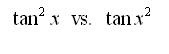In the first case we taking the tangent then squaring result and in the second we are squaring the x then taking the tangent.
The tan x^2 is actually not the best notation for this type of problem. We really should probably use tan( x^2) to make things clear.
Another thing to always keep in mind is that -1 in the cos^-1(x) is NOT an exponent , it is there to denote the fact that we are dealing with an inverse trig function. ie.There is another notation for inverse trig functions that avoids this problem, but it is not always used.## Wednesday, July 29, 2009

### What is a perfect number?

A perfect number is a whole number, an integer greater than zero; and when you add up all of the factors less than that number, you get that number. For example:

The factors of 6 are 1, 2, 3 and 6.
1 + 2 + 3 = 6

The factors of 28 are 1, 2, 4, 7, 14 and 28.
1 + 2 + 4 + 7 + 14 = 28

The factors of 496 are 1, 2, 4, 8, 16, 31, 62, 124, 248 and 496.
1 + 2 + 4 + 8 + 16 + 31 + 62 + 124 + 248 = 496

The factors of 8128 are 1, 2, 4, 8, 16, 32, 64, 127, 254, 508, 1016, 2032, 4064 and 8128.

The first four perfect numbers were known over 2,000 years ago. Some ancient cultures gave mystic interpretations to numbers that they thought were magic. Using a computer program, eventually ran for a billion of time the following 5 perfect numbers can be found:

6 = 1+2+3
28 = 1+2+4+7+14
496 = 1+2+4+8+16+31+62+124+248
8,128 = 1+2+4+8+16+32+64+127+254+508+1016+2032+4064

All of the perfect numbers that have been found so far fit the formula

2^(n-1) * ( 2^n - 1 )

where "n" is one of a very short list of prime numbers that can be used to create "Mersenne" prime numbers.

2^1 * ( 2^2 - 1 ) = 2 * 3 = 6
2^2 * ( 2^3 - 1 ) = 4 * 7 = 28
2^4 * ( 2^5 - 1 ) = 16 * 31 = 496
2^6 * ( 2^7 - 1 ) = 64 * 127 = 8,128
2^12 * (2^13-1) = 4096 * 8191 = 33,550,336
2^16 * (2^17-1) = 65536 * 131071 = 8,589,869,056
2^18 * (2^19-1) = 262144 * 524287 = 137,438,691,328
2^30 * (2^31-1) = 1073741824*2147483647=2,305,843,008,139,952,128

How many perfect numbers are there?

We do not know how many perfect numbers there are. We do know that there are an infinite number of prime numbers, which means there is a very high chance that there are an infinite number of perfect numbers. This is because there is a strong link between perfect numbers and a certain kind of prime number (the Mersenne primes).

How many perfect numbers are known?

So far, according to the Mersenne organization, there are 37 known Mersenne prime numbers. This means that there are 37 known "perfect" numbers. The newest prime number was discovered on January 27, 1998. Obviously, this is not all of them. There are many that we will never know. We don't even know if the 37th number found is the 37th number; there may be another perfect number between the 35th and the 36th. It is very likely, too, that there are many more that we will NEVER know.

Are there any odd perfect numbers?

Nobody has found any odd perfect numbers, but we do not know if any odd ones exist. If any odd perfect numbers exist, they are not based on the "Mersenne" method of calculating perfect numbers.

### What is pi?

By definition, pi is the ratio of the circumference of a circle to its diameter. Pi is always the same number, no matter which circle you use to compute it. For the sake of usefulness people often need to approximate pi. For many purposes you can use 3.14159, which is really pretty good, but if you want a better approximation you can use a computer to get it. Here's pi to many more digits: 3.14159265358979323846.

A very brief history of pi:
Pi is a very old number. We know that the Egyptians and the Babylonians knew about the existence of the constant ratio pi, although they didn't know its value nearly as well as we do today. They had figured out that it was a little bigger than 3; the Babylonians had an approximation of 3 1/8 (3.125), and the Egyptians had a somewhat worse approximation of 4*(8/9)^2 (about 3.160484), which is slightly less accurate and much harder to work with. The modern symbol for pi was first used in our modern sense in 1706 by William Jones. It(rather than some other Greek letter like Alpha or Omega) was chosen as the letter to represent the number 3.141592... because the letter [pi] in Greek, pronounced like our letter 'p', stands for 'perimeter'.
Pi is an infinite decimal. Unlike numbers such as 3, 9.876, and 4.5, which have finitely many nonzero numbers to the right of the decimal place, pi has infinitely many numbers to the right of the decimal point. If you write pi down in decimal form, the numbers to the right of the 0 never repeat in a pattern. Some infinite decimals do have patterns - for instance, the infinite decimal .3333333... has all 3's to the right of the decimal point, and in the number .123456789123456789123456789... the sequence 123456789 is repeated. However, although many mathematicians have tried to find it, no repeating pattern for pi has been discovered - in fact, in 1768 Johann Lambert proved that there cannot be any such repeating pattern. As a number that cannot be written as a repeating decimal or a finite decimal (you can never get to the end of it) pi is irrational: it cannot be written as a fraction (the ratio of two integers).
Pi shows up in some unexpected places like probability and the 'famous five' equation connecting the five most important numbers in mathematics, 0, 1, e, pi , and i: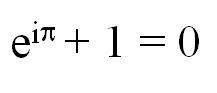## Monday, July 27, 2009

### Common Math Mistake 7

Most trig classes that I’ve seen taught tend to concentrate on doing things in degrees. I suppose that this is because it’s easier for the students to visualize, but the reality is that almost all of calculus is done in radians and students too often come out of a trig class ill prepared to deal with all the radians in a calculus class.
You simply must get used to doing everything in radians in a calculus class. If you are
asked to evaluate cos (x) at x =10 we are asking you to use 10 radians not 10 degrees!
The answers are very, very different! Consider the following,

cos (10) = - 0.839071529076 in radians
cos (10) = 0.984807753012 in degrees

You’ll notice that they aren’t even the same sign!
So, be careful and make sure that you always use radians when dealing with trig functions in a trig class.

### POLYOMINO

A polyomino is a polygon made up of squares joined edge-to-edge. There is only one type of domino (two squares) and two types of trominoes (3 squares), but there are five different tetrominoes.

So... now take out your pen and paper to draw all the possible 35 Hexomino! Its really interesting...

## Sunday, July 26, 2009

### Convert 1 to 9 = 100

Convert 1 to 9 = 100
Convert the numbers 1 to 9 which will results to "100", by inserting any of arithmetic operations (+ - * /) into the string of above numbers.
And one more interesting thing is by keeping the numbers in the above order i.e. 123456789..

One is: 1+2+3-4+5+6+78+9

Some others are:

123+45-67+8-9=100

12+3+4-56/7+89=100

1+2+3+4+5+6+7+8*9=100

-1 + (2*3*4) + (5*6)+(7*8) - 9 =100

1*2+3+45+67-8-9=100

(1+2+3+4)*5+67-(8+9)=100

If u have any other such expression, please write in the comment ....

### TRICK TO SUBSTRACT NUMBER EASILY

This is an easy trick
To subtract a large number from 1,000
subtract all but the last number from 9, then subtract the last number from 10

1000
-547

step1: subtract 5 from 9 = 4
step2: subtract 4 from 9 = 5
step3: subtract 7 from 10 = 3

### BEAUTY OF MATHS

1 x 8 + 1 = 9
12 x 8 + 2 = 98
123 x 8 + 3 = 987
1234 x 8 + 4 = 9876
12345 x 8 + 5 = 98765
123456 x 8 + 6 = 987654
1234567 x 8 + 7 = 9876543
12345678 x 8 + 8 = 98765432
123456789 x 8 + 9 = 987654321

1 x 9 + 2 = 11
12 x 9 + 3 = 111
123 x 9 + 4 = 1111
1234 x 9 + 5 = 11111
12345 x 9 + 6 = 111111
123456 x 9 + 7 = 1111111
1234567 x 9 + 8 = 11111111
12345678 x 9 + 9 = 111111111
123456789 x 9 + 10 = 1111111111

9 x 9 + 7 = 88
98 x 9 + 6 = 888
987 x 9 + 5 = 8888
9876 x 9 + 4 = 88888
98765 x 9 + 3 = 888888
987654 x 9 + 2 = 8888888
9876543 x 9 + 1 = 88888888
98765432 x 9 + 0 = 888888888

1 x 1 = 1
11 x 11 = 121
111 x 111 = 12321
1111 x 1111 = 1234321
11111 x 11111 = 123454321
111111 x 111111 = 12345654321
1111111 x 1111111 = 1234567654321
11111111 x 11111111 = 123456787654321
111111111 x 111111111 = 12345678987654321

12345679 x 9 = 111111111
12345679 x 18 = 222222222
12345679 x 27 = 333333333
12345679 x 36 = 444444444
12345679 x 45 = 555555555
12345679 x 54 = 666666666
12345679 x 63 = 777777777
12345679 x 72 = 888888888
12345679 x 81 = 999999999

9 x 9 = 81
99 x 99 = 9801
999 x 999 = 998001
9999 x 9999 = 99980001
99999 x 99999 = 9999800001
999999 x 999999 = 999998000001
9999999 x 9999999 = 99999980000001
99999999 x 99999999 = 9999999800000001
999999999 x 999999999 = 999999998000000001

6 x 7 = 42
66 x 67 = 4422
666 x 667 = 444222
6666 x 6667 = 44442222
66666 x 66667 = 4444422222
666666 x 666667 = 444444222222
6666666 x 6666667 = 44444442222222
66666666 x 66666667 = 4444444422222222
666666666 x 666666667 = 444444444222222222

111/1+1+1 = 37
222/2+2+2 = 37
333/3+3+3 = 37
444/4+4+4 = 37
555/5+5+5=37
666/6+6+6=37
777/7+7+7=37
888/8+8+8=37
999/9+9+9=37

### PLATONIC SOLIDS

Definition REGULAR POLYHEDRA:
A 3-dimensional object bounded by regular polygons.
Can you guess how many regular polyhedra exist? SURPRISINGLY…. There are only FIVE regular solids composed of just 3 regular polygons: the triangle, square and pentagon.
The five regular polyhedrons are Tetrahedron, Cube, Octahedron, Icosahedron and Dodecahedron.
Also, if you combine 2 regular polygons triangle with square, pentagon with triangle and so on, you can make other polyhedra. There are 13 such more solids known as “Archimedian Solids”.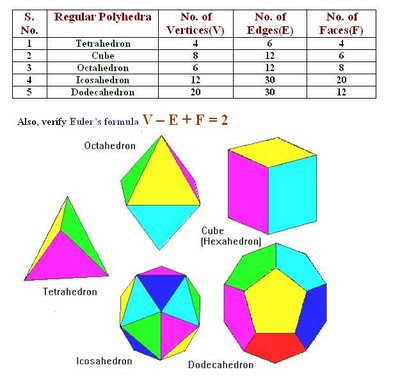### Ramanujan Number 1729

One favorite story about Ramanujan revolves around a visit that Hardy paid to him in hospital. Hardy and Ramanujan had a habit of discussing the properties of different numbers. On this particular visit, Hardy commented to Ramanujan that the number of the taxi that he had just arrived in was 1729 -- a very uninteresting number. Ramanujan quickly replied that it was in fact a very interesting number as it was the smallest number that could be represented as the sum of two cubes in two ways:

1729 = 103 + 93
and
1729 = 123 + 13

### Common Math Mistake 6

What is the value of given expression?
Many a times while solving some question, we have division of two fractions.
For example: simplifyThis has got two possible values… HOW? Check this out!Noticed the difference in the way of solving! Well, which is the correct answer? BOTH...
In case (i) it is divide 2 by (3/5) and in case (ii) it is divide (2/3) by 5 .
In doubt? Do comment!

## Saturday, July 25, 2009

### Common Math Mistake 5

Distributive Property of multiplication over addition

Let me consider a very common mistake committed by many students. Simplify the following:Many students usually simplify this as

Do not forget to apply the distributive property ie. 3 will get multiplied with both 2x and 5. So, the correct answer is:

Hope you will not be one of them …

## Friday, July 24, 2009

### Common Math Mistake 4

Proper Use of Square Root
There seems to be a very large misconception about the use of square roots out there.
Students seem to be under the misconception thatThis is not correct however. Square roots are ALWAYS positive! So the correct value isThis is the ONLY value of the square root! If we want the -4 then we do the following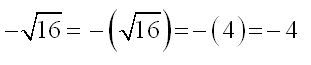Notice that I used parenthesis only to make the point on just how the minus sign was appearing! In general the middle two steps are omitted. So, if we want the negative value we have to actually put in the minus sign!

Here is the proper solution technique for this problem.Note that the ± shows up in the second step before we actually find the value of the square root! It doesn’t show up as part of taking the square root.

So remember that square roots ALWAYS return a positive answer. If you want a negative you’ll need to put it in a minus sign BEFORE you take the square root.

## Thursday, July 23, 2009

### Common Math Mistake 3



Consider this question: Solve the following for x :Too many students get used to just canceling (i.e. simplifying) things to make their life easier. So, the biggest mistake in solving this kind of equation is to cancel an x from both sides to get,While,x = 1 is a solution, there is another solution that we’ve missed. Can you see what it is?
Here’s the correct way to solve this equation. First get everything on one side then factor!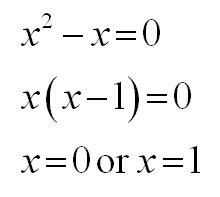Clearly x = 0 will work in the equation and so is a solution!

## Wednesday, July 22, 2009

### 22 July 2009 is Pi Approximation Day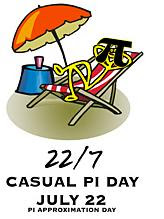Dear all
Today is Pi Approximation Day. Pi Day and Pi Approximation Day are two unofficial holidays held to celebrate the mathematical constant pi. Pi Day is observed on March 14 (3/14 in American date format) due to pi being roughly equal to 3.14. Sometimes it is celebrated more specifically on March 14 at 1:59 p.m. (commonly known as the “Pi Minute”). If pi is rounded out to seven decimal places, it becomes 3.1415926, making March 14 at 1:59:26 p.m. the “Pi Second.” Pi Approximation Day may be observed on any of several dates, most often July 22 (22/7 in European date format is a popular approximation of pi). The first Pi Day celebration was held at San Francisco's Exploratorium in 1988, with staff and the public marching around one of its circular spaces and then consuming fruit pies. The Massachusetts Institute of Technology often mails out its acceptance letters to be delivered to prospective students on Pi Day.

### Common Math Mistake 2

One common error that many students make is based on what they assume.
Since 2(x + y) = 2x + 2y then everything works like this. However, here is a whole list in which this doesn’t work.It’s not hard to convince yourself that any of these aren’t true. Just pick a couple of numbers and plug them in! For instance,I hope that you will surly not commit this error!

## Tuesday, July 21, 2009

### Bihar cop in league of top mathematicians

An ADG-rank IPS officer of Bihar Amrik Singh Nimbran has joined the select league of world's top mathematicians with his new formulae for computing the value of pi' having been uploaded on http://www.machination.eclipse.co.uk.
The website is jointly edited by well-known maths wizards Michael Roby Wetherfield of the UK and Prof Hwang Chien-lih of National Taiwan University, Taipei.
A collection of identities for computing the value of pi', discovered by Nimbran during 2007-08, were sent to the two mathematicians on June 1, 2009. Four of these made their way to the website on June 7, 2009. In a subsequent e-mail to Nimbran, Wetherfield acknowledged that "stimulated by your (Nimbran's) results, I generated new identities myself within a week".
Nimbran came up with two more formulae this month. These two too Wetherfield and Chien-lih uploaded on their website. "I am surprised by your super ability about finding new excellent identities!" Chien-lih wrote to Nimbran in a congratulatory mail.
Pi' is a mysterious number that denotes the ratio of the circumference of a circle to its diameter. In general parlance, its value is taken as 22/7 which is just an approximation. Mathematicians across the world have been fascinated by this number and research has been going on to find a better approximation. Therefore, any formula which gives a better value of the pi' in the shortest possible time is welcome in the mathematical world.
The Bihar cop has been engaged in mathematical research actively for over a decade and some of his research papers have been published in reputed journals brought out by Bihar Mathematical Society, Indian Mathematical Society and National Council of Educational Research and Training. His papers were also sent to the journals of London Mathematical Society and Fib Quarterly of The Fibonacci Association (USA) which commented favourably on the works of Nimbran.
A BA with economics and maths as optionals, Nimbran was the first non-mathematician to give an elementary proof of Fermat's last theorem for a cube in which he had proved that no integral cube number can be broken into two integral cube numbers. Prof K C Prasad of Ranchi University and Prof Emeritus Tej Narain Sinha of Bhagalpur University, who are regarded as the authority on Tarry-Escott problem (on numbers), expressed surprise on seeing Nimbran's proof.
"I was inspired by reading the works of great Indian mathematician S Ramanujan," Nimbran told TOI. He said he evaluates the value of pi' through different approaches using Inverse Tangent Function which was first used by John Machin in 1706 and has since been used till date to find the value of pi'.
"Now there are only three leading authorities on pi' in the world --Wetherfield, Lih and Jorg Arndt of Australian National University," Nimbran said. He was modest enough not to include his name in the league.

### Common Math Mistake 1

Division by Zero
Everyone knows that 0/2 = 0 , the problem is that far too many people also say that 2/0 = 0 or 2/0= 2! Remember that division by zero is undefined! You simply cannot divide by zero so don’t do it!
Here is a very good example that can arise when you divide by zero. See if you can find the mistake in the work below.So, we’ve managed to prove that 1 = 2! Now, we know that’s not true so clearly we made a mistake somewhere. Can you see where the mistake was made?
The mistake was in step 5. Recall that we started out with the assumption a = b .
However, if this is true then we have a -b = 0 ! So, in step 5 we are really dividing by zero!
That simple mistake led us to something that we knew wasn’t true, however, in most cases your answer will not obviously be wrong. It will not always be clear that you are dividing by zero, as was the case in this example. You need to be on the lookout for this kind of thing.
Remember that you CAN’T divide by zero!

### Math is NOT a spectator sport!

Dear Students
I see too many students trying to do the bare minimum required to pass the class, or at least what they feel is the bare minimum required. The problem with this is they often underestimate the amount of work required early in the class, get behind, and then spend the rest of the session playing catch up and having to do far more than just the bare minimum.
You should always try to get the best grade possible! You might be surprised and do better than you expected. At the very least you will lessen the chances of underestimating the amount of work required and getting behind.
Remember that math is NOT a spectator sport! You must be actively involved in the learning process if you want to do well in the class.
I will now write few posts about the common math mistakes which students do commit.I’m convinced that many of the mistakes are caused by student getting lazy or getting in a hurry and not paying attention to what they’re doing.
I hope that the students will be able to understand this and will assure not to repeat such mistakes in future.

## Monday, July 20, 2009

### NUMBER OF ZEROES

Dear All
Let me share a very interesting question with all of you.
If you multiply first 100 natural numbers, how many zeroes will come at the end of this product?
Do we really need to multiply all the numbers from 1 to 100 or else you have some logic?well, class XI and XII students know well about the greatest integer function. Junior class students can also find out a simple logic to solve this problem.

## Sunday, July 19, 2009

### Kaprekar Constant

Dear All

Given by D.R. Kaprekar (1905-1988) : Fond of numbers. Well known for "Kaprekar Constant" 6174.
Procedure to arrive Kaprekar Constant:
1. Take any four digit number in which all digits are not alike.
2. Arrange its digits in descending order and subtract from it the number formed by arranging the digits in ascending order.
3. If this process is repeated with reminders, ultimately number 6174 is obtained, which then generates itself.

Try it! its very interesting...

## Friday, July 17, 2009

### Which is greater: pi or 22/7?

Hello all
We know that the approximate value of pi is 22/7. Have you ever thought that which is actually greater, pi or 22/7?
Here is a result from calculus (don't worry, if you have not studied that... just enjoy it!)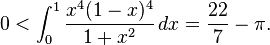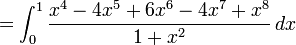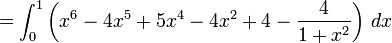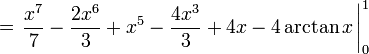Here, the given function is positive and since it is integrated in positive limits, so its value is positive.

\frac{22}{7}-\pi> 0
i.e
\frac{22}{7} > \pi`

### Divisibility rules for 7, 13, etc.

Hello all
When i was studying in the junior classes, I was taught about the divisibility rules for 2, 3, 4, 5, 6, 8, 9, 10 etc... I asked my teacher that may be by mistake you have skipped the rule for number 7. He told that there is no divisibility rule for 7. I was surprised, but no solution at that time.
Well, after many years... I came across rule for not only 7, but for given any prime number using vedic mathematics... it sounds good!
I am sharing that with all the readers.## Thursday, July 16, 2009

### No. of Squares and Rectangles

Hello all
Many a times in competitive exams the questions of counting the no. of squares and rectangles in the given arrangement comes. I am here sharing you with the direct formula for the same.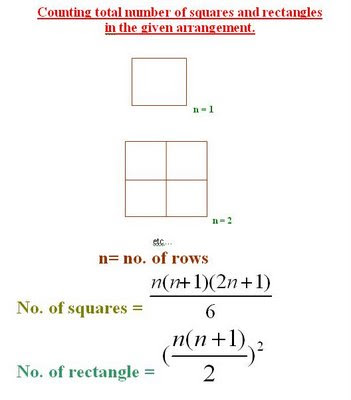Hope you have enjoyed this!

## Wednesday, July 15, 2009

Today there was a final match basketball (Sr.) between Shakti and Shram house. Both the teams played very well and finally it was Shakti house which won the match. Well... I witnessed the whole match along with my class XI C (no teaching in XI C today). The weather was quite pleasant today... I really enjoyed the match.

## Tuesday, July 14, 2009

### XII C teaching

The concept of differentiation was introduced in the class XII. Various formulas (some with proof) were considered in the class. Please solve the following questions:Click the above image for better view.

## Monday, July 13, 2009

### Fermat's Last Theorem

In the margin of his copy of a book by Diophantus, Pierre de Fermat wrote that it is possible to have a square be the sum of two squares, but that a cube can not be the sum of two cubes, nor a fourth power be a sum of two fourth powers, and so on. Further, he wrote that he had found a truly marvelous proof which the margin was too small to contain.
Fermat's Last Theorem states that
x^n + y^n = z^n
has no non-zero integer solutions for x, y and z when n > 2.
That is to say, there are no integers x, y, z such that x^3 + y^3 = z^3, or integers x, y, z such that x^7 + y^7 = z^7.
Although this is easily stated, it has proved to be one of the most puzzling problems in the whole history of mathematics. Long after all the other statements made by Fermat had been either proved or disproved, this remained; hence it is called Fermat's Last Theorem (actually, Conjecture would be more accurate than Theorem).
This conjecture was worked on by many famous mathematicians. Fermat himself proved this theorem for n = 4, and Leonhard Euler did n = 3. Special cases were dispatched one after another. New theories were developed to attack the problem, but all attempts at a general proof failed. They failed, that is, until this decade, when, building on work of many famous mathematicians, Prof. Andrew Wiles of Princeton University finally proved it in 1996. His method could not have been known to Fermat. Fermat's "truly marvelous proof" is now believed to have been faulty.
The actual proof is very indirect, and involves two branches of mathematics which at face value appear to have nothing to do either with each other or with Fermat's theorem. The two subjects are elliptic curves and modular forms and involve work done previously by Taniyama and Shimura. The greatest difficulty was in proving that the Taniyama-Shimura conjecture was true. This is the contribution made by Andrew Wiles, and the final stage in establishing Fermat's Last theorem.

### Inspiring Math Quotations

"The length of your education is less important than its breadth, and the length of your life is less important than its depth." -- Marilyn Savant

"Perfect numbers like perfect men are very rare." – Descartes

"The reading of all good books is like a conversation with the finest people of past centuries." – Descartes

"I hear and I forget. I see and I remember. I do and I understand." -- Chinese Proverb.

Small minds discuss persons. Average minds discuss events. Great minds discuss ideas. Really great minds discuss mathematics."

"The advancement and perfection of mathematics are intimately connected with the prosperity of the state." – Napoleon

"A man is like a fraction whose numerator is what he is and whose denominator is what he thinks of himself. The larger the denominator, the smaller the fraction." – Tolstoy

"The mathematical sciences particularly exhibit order, symmetry, and limitations; and these are the greatest forms of the beautiful." – Aristotle

### NUMBER OF TRAILING ZEROES IN A PRODUCT (PART -2)

Dear all, Please do watch my latest video (dated 10-01-2021) on the topic “NUMBER OF TRAILING ZEROES IN A PRODUCT (PART -2)” VIDEO LINK HERE...